Need Help? Chat with us

# Find Function in Excel | Excel Formulas in Hindi

## Find Function in Excel

Find function is one of the many functions that are available in Excel. Find function is used to return the position of a specific character or string within a text range. Excel Find function returns the number of one text string inside another.

Find function is categorized under the head String/Text function. It is an in-built function in Excel. It is a part of the formula so it can be used as a worksheet function. The find function is case sensitive.

## Special Offer for First Time Users

If you are the first time user and want to learn Microsoft office skills, then you can avail this special offer discount on your email.In case if you want to search for non-case-sensitive, you can use the Search Function in Excel.

The Search and Find function are almost similar to each other. To find the position of the sub string in the string we use the Find function. It will help you to find the location of text in the string. It returns numeric value.

Find function was introduced in the year 2000. and learn more Function at Excel Functions in Hindi

Note: The function does not allow use of wildcard characters. Whereas, Search function do allow use of wildcard character.

# The Syntax of the Find Function in Excel

FIND(find_text, within_text, [start_num])

Where,

Find_text: This is the argument entered to find the character or sub string. This argument is compulsory

Within_text: This is the argument to enter the String we want to search within. This argument is compulsory.

Start_num: This is an option argument which specifies the search cell range. It you do not enter it starts the search from the 1st character of the within_text range.

Note: If the function does not find the find text characters it will return #VALUE!

This function would be clearer when you go through the examples below. Below are the different examples, each examples shows how the find function works in Excel. Let’s take a look on 5 examples one by one.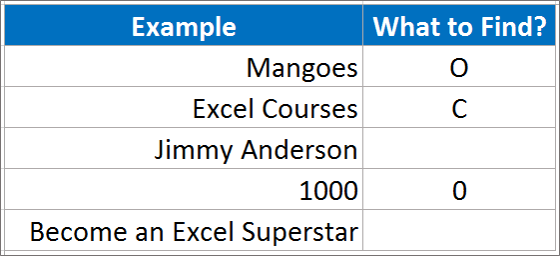Example 1: Mangoes

Here we want to find “O” from the example Mangoes. You can see in the example that we want to find O in cell C4 from the cell B4. Enter the find Formula and Press Enter

Result: What do we see? #Value. Now you would ask why error. This is because you entered Uppercase O instead of lowercase o. As in the example Mangoes there is no Uppercase O.

So, it is to be noted that you will only find the exact match available and so match and find function are very similar.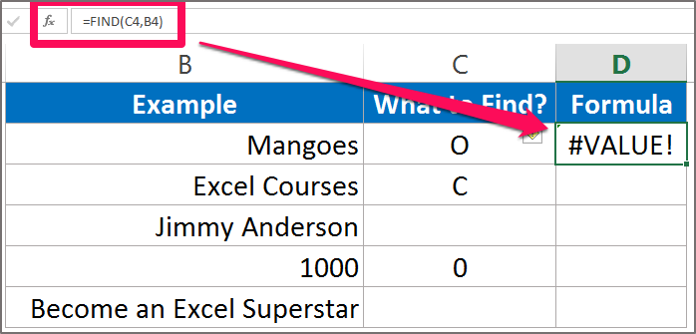Example 2: Excel Course

Here we want to find “C” from the example Excel Course. You can see in the example that we want to find C in the cell C5 from the cell B5. Enter the Find formula and Press Enter.

Result: What do you see? 7 is the answer. This means that the letter C is in the 7th place in the cell B5. It also counts the space between Excel Course.

So, it is notes that the space is calculated while calculating find formula in Excel.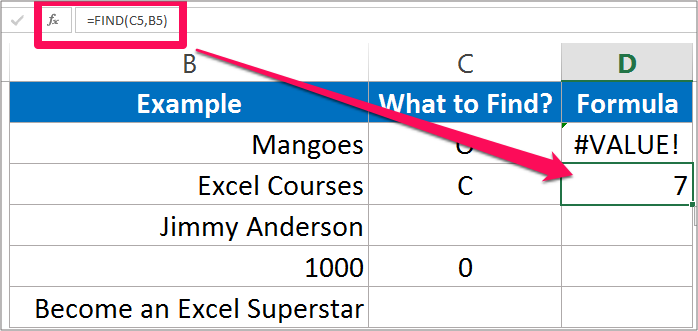Example 3: Jimmy Anderson

Here I want to find where space located in the text string is. So I will type space in the cell C6 and we want to find the space from the cell B6. Enter the Find Formula and Press Enter

Result: What did you find? It returned 6 in the result column. Why 6? It is because space is entered after the word Jimmy which is in the 6th place in the text string.

However, if you would have entered space before the word Jimmy it would return 1 as the result because it is at the 1st place in the string.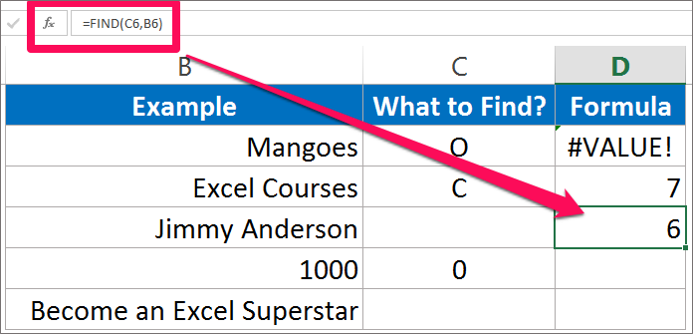Example 4: 1000

Now we take a number into consideration. Suppose we took 1000 in cell B7 and we want to find 0. Enter the Find formula and press enter.

Result: What do we get the answer? The answer is 2. This is because 0 is in the second place in the cell B7. There are three 0 in the string. So you could conclude that the answer should be 3 and 4 as well.

This function lets you find the first place where 0 is located in the string and that first place is 2nd.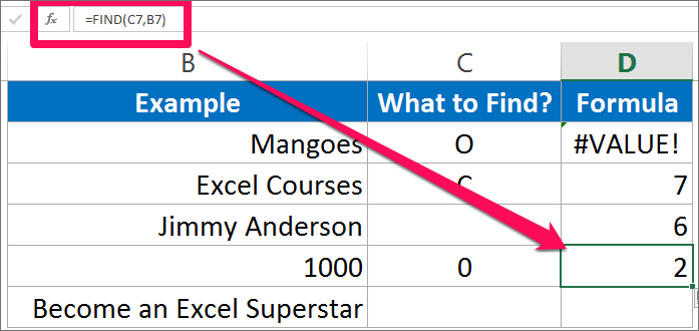Example 5: Become an Excel Superstar

Here I have left what to find function blank. C8 is empty it does not contain space either. Enter the Find function and press Enter.

Result: What do you see? The answer is 1. It turned out to be 1 as the cell contains nothing in it. If you would have entered space it would return 7. If you entered Excel it would return 11 as Excel world is at the 11th place in the string.

If you enter any wild character it would return #VALUE!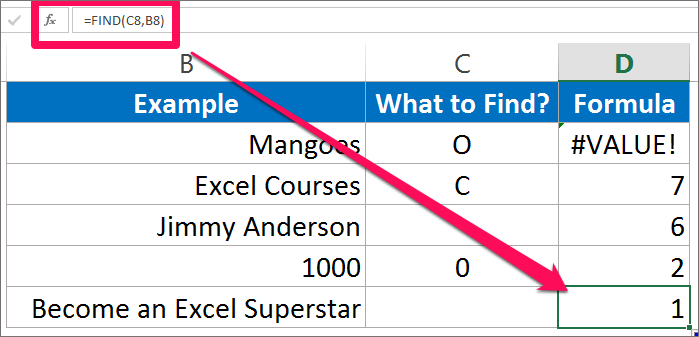The examples above have explained you regarding the find function in Excel. The possible answers for it and also how to use the find function in Excel.

Hope you like our tutorial for more cool and amazing trick of excel Like our Excel Superstar Facebook Page and subscribe to our Excel Superstar YouTube channel. Excel Superstar is the leading online training company, which provides Online Excel Course in Hindi. Connect with us and become an Excel Superstar.